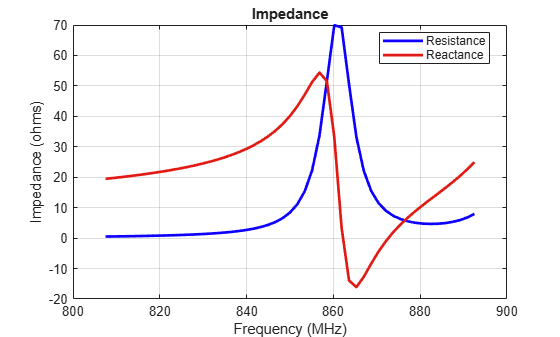# Design Variations On Microstrip Patch Antenna Using PCB Stack

Use the `pcbstack` to design basic, parasitic, direct-coupled, and CP patch antennas.

Setup parameters.

```vp = physconst('lightspeed'); f = 850e6; lambda = vp./f;```

### Design Basic Patch Antenna

Set the length and width of the patch and the groundplane.

```Lp = lambda(1)/2; Wp = lambda(1)/2; Lgp = 0.75.*lambda(1); Wgp = 0.75.*lambda(1); h = 2.e-3; p1 = antenna.Rectangle('Length',Lp,'Width',Wp,'NumPoints',30); p2 = antenna.Rectangle('Length',Lgp,'Width',Wgp); d1 = dielectric('Air');```

Define the properties of the PCB stack.

```basicPatch = pcbStack; basicPatch.Name = 'Basic Patch'; basicPatch.BoardThickness = h; d1.Thickness = h; basicPatch.BoardShape = p2; basicPatch.Layers = {p1,d1,p2}; basicPatch.FeedLocations = [-lambda(1)/8 0 1 3]; figure show(basicPatch)```Plot the impedance of the basic patch antenna.

```freq1 = linspace(f(1)-0.05*f(1),f(1) + 0.05*f(1),51); figure impedance(basicPatch,freq1)```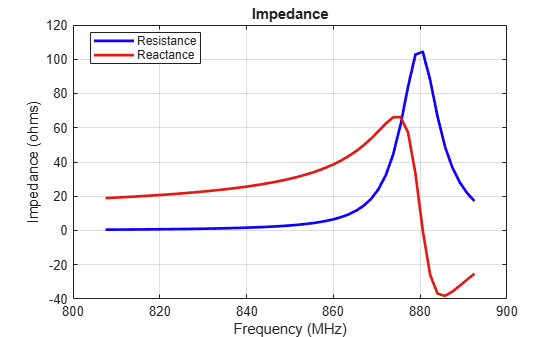Plot the radiation pattern of the basic patch antenna.

```figure pattern(basicPatch,f(1))```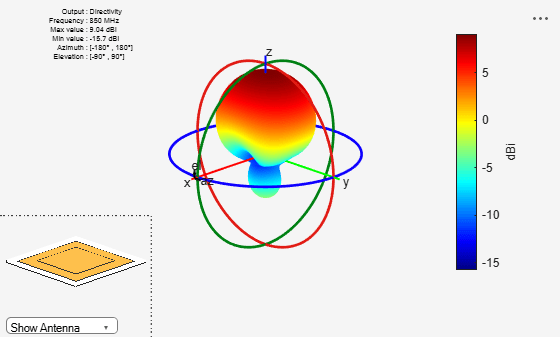### Design Parasitic Patch Antenna

Set the dimensions for the patch.

```L = 0.15; W = 1.5*L; stripL = L; gapx = .015; gapy = .01; r1 = antenna.Rectangle('Center',[0,0],'Length',L,'Width',W); r2 = antenna.Rectangle('Center',[L/2+stripL/2+gapx,0],'Length',stripL,'Width',W,'NumPoints',[2 20 2 20]); r3 = antenna.Rectangle('Center',[-L/2-stripL/2-gapx,0],'Length',stripL,'Width',W,'NumPoints',[2 20 2 20]); r = r1+r2+r3; figure show(r)```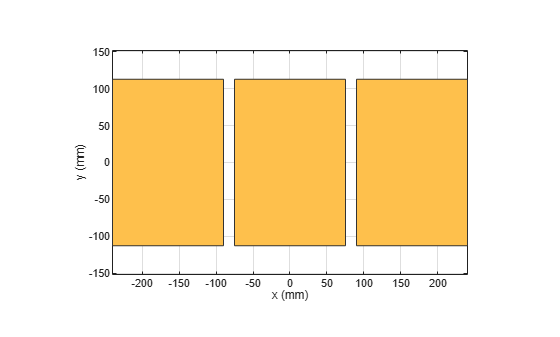Set the dimensions of the groundplane.

```Lgp = 0.55; Wgp = 0.4; g1 = antenna.Rectangle('Center',[0,0],'Length',Lgp,'Width',Wgp);```

Define the properties of the PCB stack. Create a pcb stack by driving the center radiator.

```parasitic_patch = pcbStack; parasitic_patch.BoardShape = g1; parasitic_patch.BoardThickness = .007; parasitic_patch.Layers = {r,g1}; parasitic_patch.FeedLocations = [(L)/4 0 1 2]; figure show(parasitic_patch)```Plot the S-parameters of the parasitic patch antenna.

```s = sparameters(parasitic_patch,linspace(0.8e9,1e9,11)); figure rfplot(s)```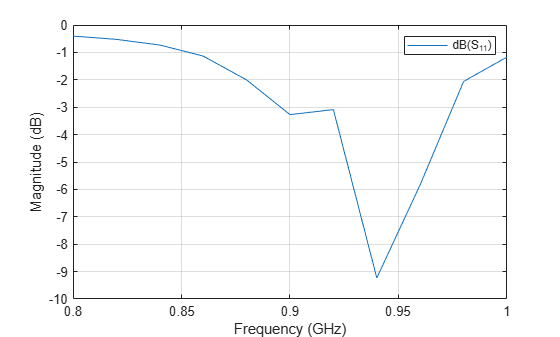Plot the radiation pattern of the parasitic patch antenna.

```figure pattern(parasitic_patch,0.896e9)```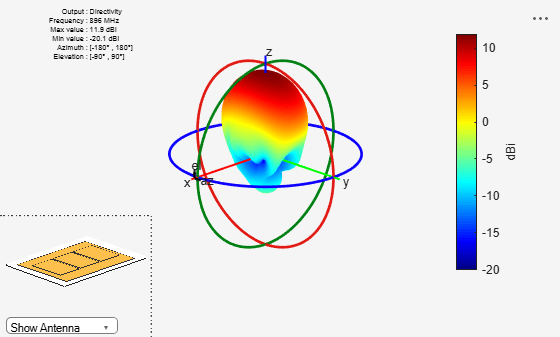### Design Direct-coupled Patch Antenna

```r2 = copy(r1); r2.Center = [lambda/1.25,0]; r3 = copy(r1); r3.Center = [-lambda/1.25,0]; r = r1+r2+r3; figure show(r)```Strip join the sections.

```r4 = antenna.Rectangle('Length',0.65*lambda,'Width',0.02*lambda,'Center',[lambda/2,0],'NumPoints',[20 2 20 2]); r5 = copy(r4); r5.Center = [-lambda/2,0]; s = r + r4 + r5; figure show(s)```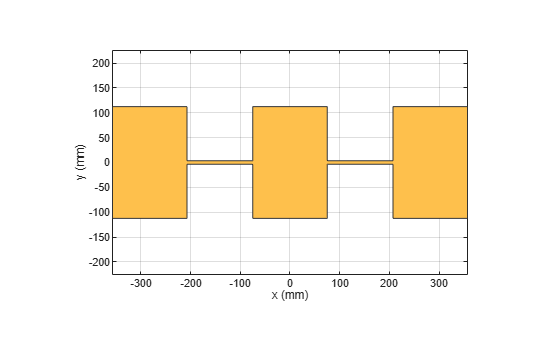Define the properties of the PCB stack.

```g1.Length = 0.8; series_patch = pcbStack; series_patch.BoardShape = g1; series_patch.Layers = {s,g1}; series_patch.FeedLocations = [L/4 0 1 2]; figure show(series_patch)```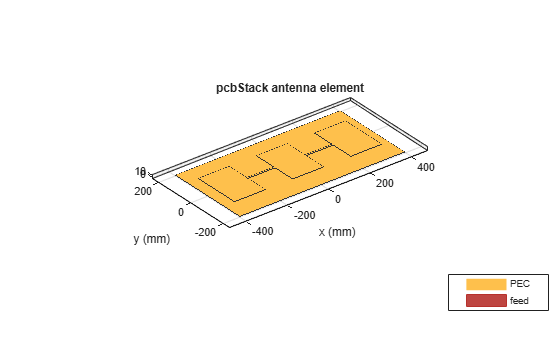Plot the radiation pattern at 1 GHz for the direct-coupled patch antenna.

```figure pattern(series_patch,1e9)```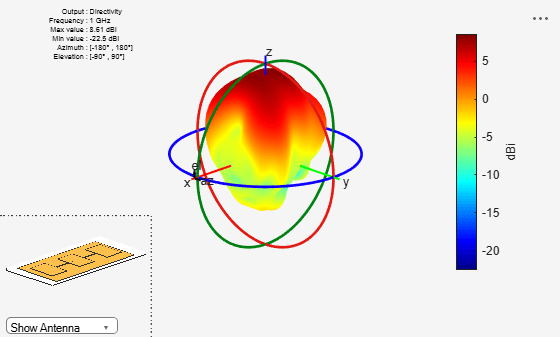Mesh the antenna using maximum edge length of 0.03 m. Plot the impedance of the direct-coupled patch antenna for the frequency range, 0.8 GHz to 1.2 GHz.

```figure mesh(series_patch,'MaxEdgeLength',0.03)```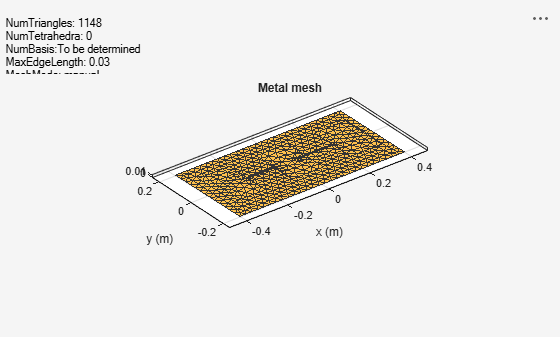```figure impedance(series_patch,linspace(0.8e9,1.2e9,81))```### Design Circularly Polarized Patch - Truncated Corners

Set the length and width of the patch and the groundplane.

```Lp = lambda(1)/2; Wp = lambda(1)/2; Lgp = 0.75.*lambda(1); Wgp = 0.75.*lambda(1); h = 2.e-3;```

Create the base shape for the patch.

`p1 = antenna.Rectangle('Length',Lp,'Width',Wp,'NumPoints',20);`

Truncate the corners of the rectangle.

```Lcorner = 0.25*Lp; Wcorner = 0.25*Wp; cornerCenter1 = [-Lp/2,Wp/2,0]; cornerCenter2 = [Lp/2,-Wp/2,0]; pcorner1 = antenna.Rectangle('Length',Lcorner,'Width',Wcorner); pcorner1 = rotateZ(pcorner1,45); pcorner1 = translate(pcorner1,cornerCenter1); pcorner2 = antenna.Rectangle('Length',Lcorner,'Width',Wcorner); pcorner2 = rotateZ(pcorner2,45); pcorner2 = translate(pcorner2,cornerCenter2); pradiator = p1 -pcorner1-pcorner2;```

Create the groundplane shape.

`p2 = antenna.Rectangle('Length',Lgp,'Width',Wgp);`

Define the dielectric Layer.

`d1 = dielectric('Air');`

Define the properties of the PCB stack for the circularly polarized patch.

```truncatedCornerPatch = pcbStack; truncatedCornerPatch.Name = 'Basic Patch'; truncatedCornerPatch.BoardThickness = h; d1.Thickness = h; truncatedCornerPatch.BoardShape = p2; truncatedCornerPatch.Layers = {pradiator,d1,p2}; truncatedCornerPatch.FeedLocations = [-lambda(1)/8 0 1 3]; figure show(truncatedCornerPatch)```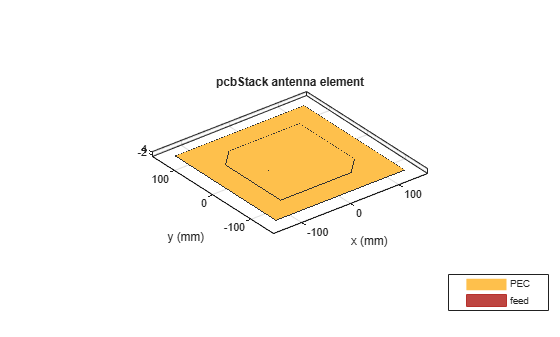Plot impedance of the circularly polarized antenna.

```figure impedance(truncatedCornerPatch,freq1)```